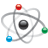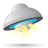# K70 DDR Configuration Issue with TWR-K70

cancel
Showing results for
Did you mean:

## K70 DDR Configuration Issue with TWR-K70

387 ViewsContributor III
Labels (2)

• ### Kinetis K Series MCUs

225 ViewsNXP TechSupport

Hi Scott,

The K70 SIM_MCR register [DDRCFG] field using to control the DDR memory types and drive strength.

The DDR_CR53-55 register [Reserved] bits field function is not visible to customer.
I do a similar test with TWR-K70F120M board, the 0x84806018 ~ 0x8480601b memory range value was modified during code operation.
For I don't know the customer application, I couldn't check if customer mentioned DDR2 memory range (0x84806018 ~ 0x8480601b) with the incorrect value.
While, if I write the fixed value to those memory range, such as 0x5555AAAA. I will read the correct value from those DDR2 memory range.
I am using below code for K70 Tower board DDR2 controller initialization.
We recommend customer to use the [Kinetis® K70 DDR memory initialization tool(REV 1.11.1)] tool:

void twr_ddr2_script_init(void)
{
/* Enable DDR controller clock */

/* Enable DDR pads and set slew rate */
SIM_MCR |= 0xC4;   // bits were left out of the manual so there isn't a macro right now

* (vuint32 *)(0x400Ae1ac) = 0x01030203;

DDR_CR00 = 0x00000400;    // DDRCLS = 4 is reserved??
DDR_CR02 = 0x02000031;
DDR_CR03 = 0x02020506;
DDR_CR04 = 0x06090202;
DDR_CR05 = 0x02020302;
DDR_CR06 = 0x02904002;
DDR_CR07 = 0x01000303;
DDR_CR08 = 0x05030201;
DDR_CR09 = 0x020000c8;
DDR_CR10 = 0x03003207;
DDR_CR11 = 0x01000000;
DDR_CR12 = 0x04920031;
DDR_CR13 = 0x00000005;
DDR_CR14 = 0x00C80002;
DDR_CR15 = 0x00000032; //  | DDR_CR15_SREF_MASK ;
DDR_CR16 = 0x00000001;
DDR_CR20 = 0x00030300;
DDR_CR21 = 0x00040232;
DDR_CR22 = 0x00000000;
DDR_CR23 = 0x00040302;
DDR_CR25 = 0x0A010201;
DDR_CR26 = 0x0101FFFF;
DDR_CR27 = 0x01010101;
DDR_CR28 = 0x00000003;
DDR_CR29 = 0x00000000;
DDR_CR30 = 0x00000001;
DDR_CR34 = 0x02020101;
DDR_CR36 = 0x01010201;
DDR_CR37 = 0x00000200;
DDR_CR38 = 0x00200000;
DDR_CR39 = 0x01010020;
DDR_CR40 = 0x00002000;
DDR_CR41 = 0x01010020;
DDR_CR42 = 0x00002000;
DDR_CR43 = 0x01010020;
DDR_CR44 = 0x00000000;
DDR_CR45 = 0x03030303;
DDR_CR46 = 0x02006401;
DDR_CR47 = 0x01020202;
DDR_CR48 = 0x01010064;
DDR_CR49 = 0x00020101;
DDR_CR50 = 0x00000064;
DDR_CR52 = 0x02000602;
DDR_CR53 = 0x03c80000;
DDR_CR54 = 0x03c803c8;
DDR_CR55 = 0x03c803c8;
DDR_CR56 = 0x020303c8;
DDR_CR57 = 0x01010002;

asm("NOP");

DDR_CR00 |= 0x00000001;

while ((DDR_CR30 & 0x400) != 0x400);

MCM_CR |= MCM_CR_DDRSIZE(1);
}

Have a great day,
Mike

-----------------------------------------------------------------------------------------------------------------------